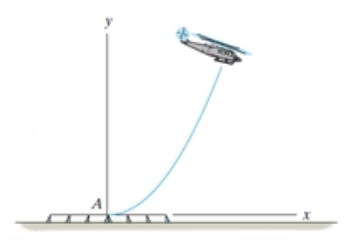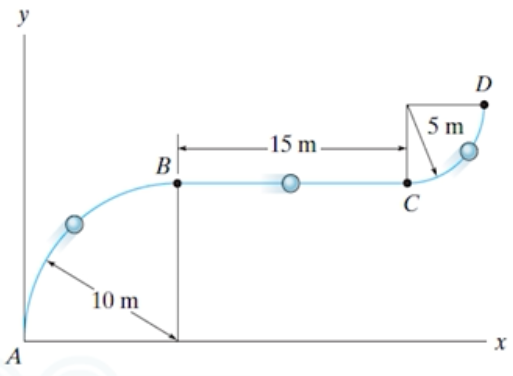Need Help?

Subscribe to Dynamic

###### \${selected_topic_name}
• Notes

$\begin{array}{l}{12-85 . \text { The flight path of the helicopter as it takes off from }} \\ {A \text { is defined by the parametric equations } x=\left(2 t^{2}\right) \mathrm{m} \text { and }} \\ {y=\left(0.04 t^{3}\right) \mathrm{m}, \text { where } t \text { is the time in seconds. Determine the }} \\ {\text { distance the helicopter is from point } A \text { and the magnitudes }} \\ {\text { of its velocity and acceleration when } t=10 \mathrm{s}}\end{array}$$x=2 t^{2} m$

$y=0.04 t^{3} \mathrm{m}$

$t=10 s \Rightarrow x=200m, y=40m \Rightarrow \Delta r=d=\sqrt {x^2+y^2}=\sqrt{(200)^2+(40)^2}\Rightarrow d=204 m$

$v_{x}=\frac{d x}{d t}=\frac{d(2 t^2)}{d t}=4 t=40 \mathrm{m} / \mathrm{s}$

$v_ y=\frac{d y}{d t}=\frac{d\left(0.04 t^{3}\right)}{d t}=0.12t^2=12 \mathrm{m} / \mathrm{s}$

$V=\sqrt{v_{x}^{2}+v_{y}^{2}}=\sqrt{4{0}^{2}+12^{2}}=41.8 \mathrm{ms}$

$a_ x=\frac{d v x}{d t}=\frac{d(4 t)}{d t}=4 \mathrm{m} / \mathrm{s}^{2}$

$a_y=\frac{d v y}{d t}=\frac{d\left(0.12 t^{2}\right)}{d t}=0.24 t=2.4 \mathrm{m} / \mathrm{s}^{2}$

$a=\sqrt{a x^{2}+a y^{2}}=\sqrt{4^{2}+2.4^{2}}$

$a=4.66 \mathrm{m} / \mathrm{s}^{2}$

$\begin{array}{l}{\text { 12- 75. A particle travels along the curve from } A \text { to } B \text { in } 2 \text { s. }} \\ {\text { It takes } 4 \text { s for it to go from } B \text { to } C \text { and then } 3 \text { s to go from } C} \\ {\text { to } D \text { . Determine its average speed when it goes from } A \text { to } D .}\end{array}$${t} _ {A \rightarrow B}=s$

$t_{B \rightarrow C}=4 S$

$t_{C \rightarrow D}=3s$

$s_{A \rightarrow B}=\frac{1}{4}(2 \pi * 10)$

$≃ 15.71 \mathrm{m}$

$S_{B \rightarrow C}=15 \mathrm{m}$

$S_{C \rightarrow D}=\frac{1}{4}(2 \pi * 5)$

$≃ 7.85$

$S_{total}=15.71+15+7 \cdot 85 ≃ 38.56 \mathrm{m}$

$V_{average \ speed}=\frac{S_ T}{t_{T}}=\frac{38.56}{2+4+3} ≃ 4.28 \mathrm{m} / \mathrm{s}$